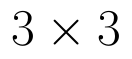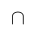Select Page

# CBSE Maths 12 Science Integrals MCQ Solutions in English

CBSE Maths 12 Science Integrals MCQ Solutions in English to enable students to get Solutions in a narrative video format for the specific question.

Expert Teacher provides CBSE Maths 12 Science Integrals MCQ Solutions through Video Solutions in English language. This video solution will be useful for students to understand how to write an answer in exam in order to score more marks. This teacher uses a narrative style for a question from Integrals not only to explain the proper method of answering question, but deriving right answer too.

Please find the question below and view the Solution in a narrative video format.

Question:

Solution Video in English:

## Similar Questions from CBSE, 12th Science, Maths, Integrals

Question 1 : Find the integral of the function. (View Answer Video)

Question 2 : Find :(View Answer Video)

Question 3 : Find:. (View Answer Video)

Question 4 : Find the integral of the function. (View Answer Video)

Question 5 : Evaluate :(View Answer Video)

### Determinant

Question 1 : Using the properties of determinants, evaluate. (View Answer Video)

Question 2 : Evaluate. (View Answer Video)

Question 3 : Let A be the non- singular square matrix of order, then |adj A| is equal to, (View Answer Video)

Question 4 : Evaluate  the determinants in :. (View Answer Video)

Question 5 : Iffind |A|. (View Answer Video)

### Application of Derivatives

Question 1 : The point on the curvewhich is nearest to the point (0, 5) is :

Question 2 : Find approximate value of. (View Answer Video)

Question 3 : Find approximate value of(View Answer Video)

Question 4 : For all real values of x the minimum value of.(View Answer Video)

Question 5 : Sand is pouring from a pipe at the rate of. The falling sand forms a cone on the ground in such a way that the height of the cone is always one-sixth of the radius of the base. How fast is the height of the sand cone increasing, when the height is 4 cm? (View Answer Video)

### Probability

Question 1 : If A and B are two events such that P(A)=0.3, P(B)=0.6 and P(B/A)=0.5, find P(AB). (View Answer Video)

Question 2 : An urn contains 4 balls. Two balls are drawn at random from the urn (without replacement) and are found to be white. What is the probability that all the four balls in the urn are white?  (View Answer Video)

Question 3 : A box contains 50 bolts and 50 nuts. Half of the bolts and nuts are rusted. If two items are drawn with replacement, what is the probability that either both are rusted or both are bolts.    (View Answer Video)

Question 4 : A bag contain 2 red, 6 black and 8 green balls. A ball is drawn at random from the bag. Find the probability of black balls:
Question 5 : Compute P(A/B) if P(B) =0.5 and P(AB)=0.32. (View Answer Video)